# HOTS: Higher-Order Thinking Skills

Develop critical thinking, connect math concepts and master problem solving with the toughest questions available in KooBits.
Loved by 200,000+ parents & students with proven results!

## Why use HOTS?

### Go beyond facts and memorisation

Effective way to expand your child’s thinking

### Build a high-demand skill

Transform your child into a lifelong learner, and prepare them for the real world

### Create a sense of achievement

HOTS questions aren’t easy. But doing them will boost your child’s motivation in math

Higher-Order Thinking Skills (HOTS) distinguishes critical thinking skills from low-order learning outcomes, such as those attained by rote memorization.

HOTS is based on various taxonomies of learning, particularly by Benjamin Bloom in 1956. The chart shows a revised version of Bloom’s taxonomy from Anderson, L. W. & Krathwohl, D.R., et al (2001).

HOTS is believed to better prepare children beyond the standard school maths curriculum, and empower them to interpret ideas, information, discover new solutions and improve their understanding in maths.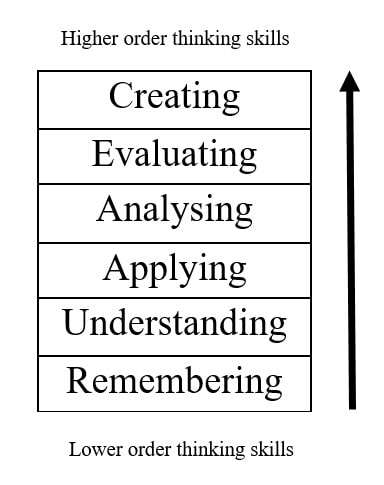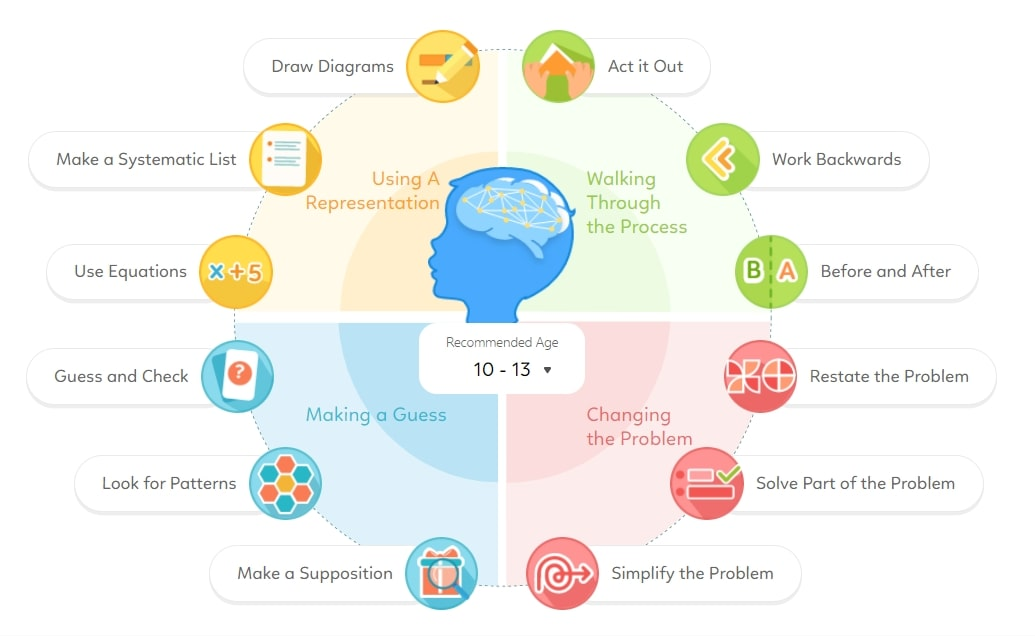Students who have good level of HOTS are expected to succeed in their studies later."
With HOTS, your child is likely to achieve:

## Let's see how HOTS makes difference

### Standard Question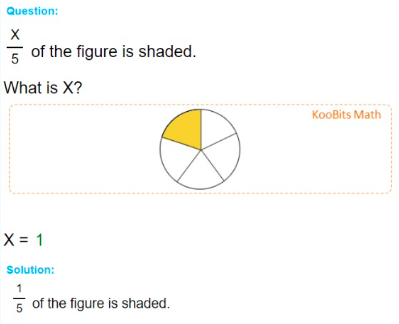### HOTS Question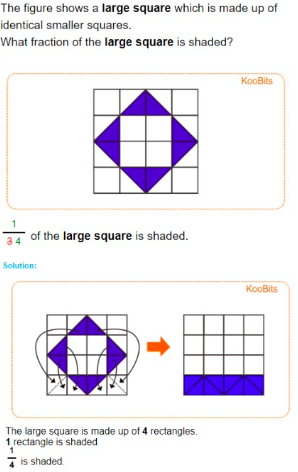HOTS questions encourage your child to explore, interpret ideas and arrive at solutions by themselves.

Every topic at every grade level is specially designed to improve problem solving skills which can then be applied to real life situations.

• Age 6 - 9
• Age 8 - 11
• Age 10 - 13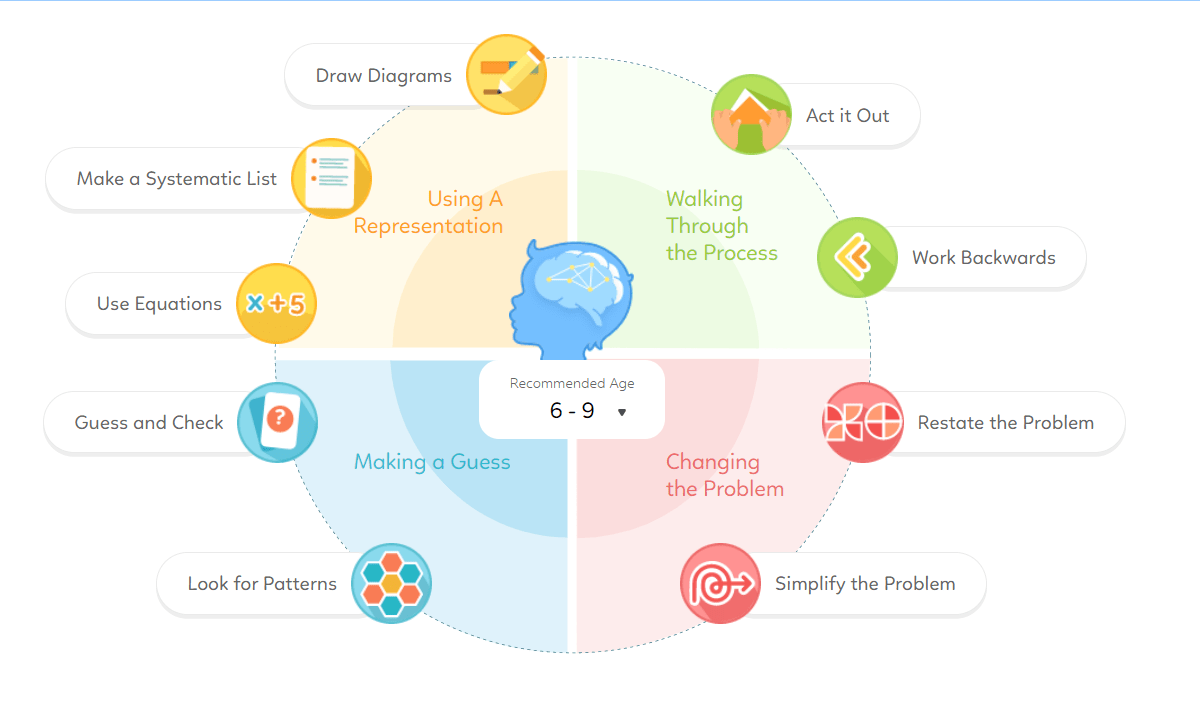Draw a Diagram
• Basic - Numbers to 10 - 1 quantity, amount of 1 quantity, quantity added
• Basic - Numbers to 10 - 2 quantities, number of ways
• Challenging - Numbers to 10 - Ordering objects/people
• Basic - Numbers to 20 - 2 quantities, 1 condition
• Basic - Numbers to 40 - 2 quantities, total quantity, amount of 1 quantity
• Basic - Numbers to 40 - 2 quantities, number of each quantity
• Intermediate - Numbers to 40 - 2 quantities, total quantity, total amount, amount of each quantity
• Basic - Number to 100 - 2 quantity, amount of 1 quantity, 1 comparison
• Basic - Numbers to 100 - Equal Stage (start), internal transfer, amount transferred
• Challenging - Whole Numbers - ordering, more than 3 comparisons
• Basic - Numbers to 1000 - 2 quantities, a common item with 2 different amount of items
• Basic - Numbers to 1000 - 2 quantities, total amount, 1 comparison
• Intermediate - Numbers to 1000 - 2 quantities, amount for each quantity
• Intermediate - Numbers to 1000 - Equal Stage (end), 2 quantities, amount added/subtracted
• Challenging - Numbers to 1000 - 3 quantities, 2 comparisons
• Challenging - Numbers to 1000 - 3 quantities, 2 comparisons, amount of 1 quantity
• Challenging - Numbers to 1000 - Equal Stage (end), internal transfer, 3 quantities, amount for each quantity
• Basic - Ordinal Numbers - 1 quantity, 2 conditions
• Challenging - Ordinal Numbers - 2 quantities, 3 or more events
• Intermediate - Multiplication & Division - cost of 1 item, number of item for each quantity, total number
• Intermediate - Multiplication & Division - 2 quantities, number in 1 set, amount of 1 quantity
• Intermediate - Length - total length, length of 1 quantity, rest are equal length
• Intermediate - Length - ordering height/length, 2 or 3 comparisons
• Challenging - Length - 2 quantities, length of each quantity measured with different items, relationship of items
• Challenging - Length - 2 quantities, total distance, 2 distances from different start point
• Intermediate - Mass - 3 quantities, total mass, 1 comparison
• Intermediate - Money - 2 quantities, total amount of a set, amount of 1 quantity
• Basic - Fractions - Fraction required, find number of parts required
Make a Systematic List
• Basic - Numbers to 10 - 1 quantity, 2 conditions
• Basic - Numbers to 10 - Number of possible pairs
• Intermediate - Numbers to 10 - 2 quantities, 2 conditions, 1 comparison
• Basic - Numbers to 20 - 2 quantities, total quantity, 1 comparison
Use Equations
• Basic - Whole Numbers - 2 quantities, different amount of quantities of same value, cost of one item
• Intermediate - Whole Numbers - 2 quantities, total amount of a set, amount of 1 quantity
Guess and Check
• Intermediate - Numbers to 100 - 2 quantities, total quantity, total amount, amount of each quantity
• Challenging - Numbers to 1000 - 3 quantities, total amount, list of possible choices
• Challenging - Money - 3 quantities, total quantity, total amount
Look for Patterns
• Basic - Numbers to 10 - Figure/ Number Pattern
• Intermediate - Numbers to 100 - Number Patterns, 2 rules
• Challenging - Numbers to 100 - Sequence Pattern, specific term
• Intermediate - Shapes - Sequence Pattern, missing pattern
• Challenging - Figure Pattern - 2 different quantities, find next pattern
Act it Out
• Intermediate - Whole Numbers - 2 conditions, number of possible ways
• Intermediate - Whole Numbers - 4 or more quantities, number of possible pairs
• Challenging - Ordinal Numbers - 3 or more quantities, 3 or more conditions
• Basic - Fractions - Shaded part of a Figure
• Basic - Shapes - 1 quantity, total quantity, arranging to form a shape
Work Backwards
• Intermediate - Numbers to 40 - 2 quantities, 2 events, final amount
• Challenging - Numbers to 40 - 3 quantities, 3 events, final amount
• Basic - Numbers to 1000 - 1 quantity, 1 or 2 event(s), amount of quantity (end)
• Intermediate - Time - End time, duration of 2 events
Restate the Problem
• Intermediate - Numbers to 100 - Sum and subtract 3 numbers
• Challenging - Numbers to 100 - Sum and subtract more than 3 numbers
• Basic - Numbers to 1000 - Sum of 2 numbers
• Basic - Numbers to 1000 - Subtraction of 2 numbers
• Basic - Numbers to 40 - sum up to 10 numbers
• Intermediate - Numbers to 100 - 2 quantities, 2 equations
• Challenging - Whole Numbers - Equal Stage (end), internal transfer, 3 quantities, amount of each quantity
• Intermediate - Mass - 2 quantities, different amount of quantities of same mass, amount of 1 quantity
• Challenging - Picture graphs - Complete picture graphs with scale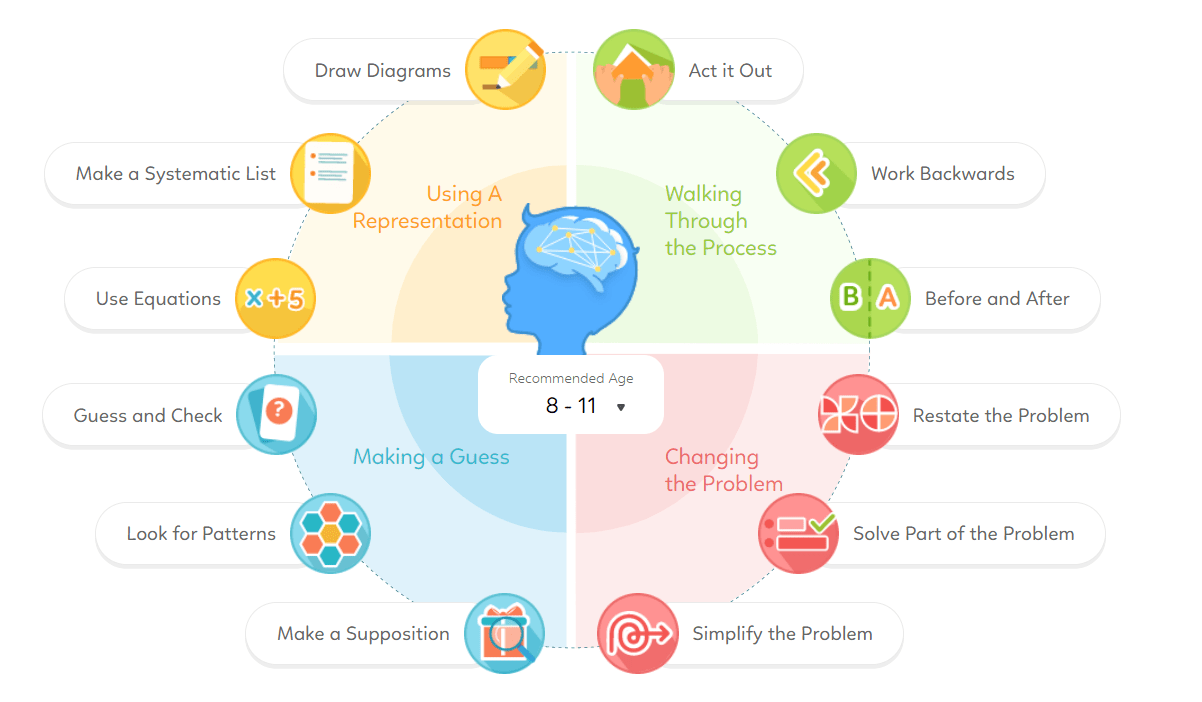Draw a Diagram
• Basic - Whole Numbers - internal transfer, 2 quantities, 1 comparison, amount transferred
• Basic - Whole Numbers - Internal transfer, 2 quantities, total amount, amount transferred
• Intermediate - Whole Numbers - 2 quantities, total amount of 2 sets
• Intermediate - Whole Numbers - Equal Stage (end), everything changed, 2 quantities, total amount
• Intermediate - Whole Numbers - Equal Stage (end), everything changed, 2 quantities, 1 relationship
• Intermediate - Whole Numbers - Constant quantity, 2 quantities, 1 comparison, 1 relationship, amount added/subtracted
• Intermediate - Whole Numbers - Internal transfer, 2 quantities, 1 comparison, 1 relationship, amount transferred
• Intermediate - Whole Numbers - Internal transfer, 2 quantities, 2 relationships, amount transferred
• Challenging - Whole Numbers - 3 quantities, 3 different characteristics, overlap in characteristics
• Challenging - Whole Numbers - 3 quantities, 2 comparisons, total amount
• Challenging - Whole Numbers - total amount, amount of 1 quantity, rest are equal
• Challenging - Whole Numbers - 3 quantities, 2 relationships, amount of 1 quantity
• Challenging - Whole Numbers - 3 quantities, total amount, 1 comparison
• Challenging - Whole Numbers - 3 quantities, 2 relationships, total amount
• Challenging - Whole Numbers - 3 quantities, total amount, amount of 2 quantities, 1 relationship
• Challenging - Whole Numbers - Internal transfer, 2 quantities, 1 comparison, 2 relationships, amount added/subtracted
• Basic - Multiplication & Division - Grouping, 1 quantity, amount of a group, total amount
• Basic - Multiplication & Division - 2 quantities, total amount of a set, 1 comparison
• Basic - Multiplication & Division - Internal transfer, 2 quantities, 2 comparisons
• Intermediate - Multiplication & Division - Constant quantity, 2 quantities, 2 relationships, amount changed
• Intermediate - Multiplication & Division - Grouping, 2 quantities, amount of a set, total amount
• Challenging - Multiplication & Division - Equal Stage (start), everything changed, 2 quantities, 1 relationship
• Basic - Fractions - 2 quantities, fraction for each quantity, 2 wholes, compare fractions
• Basic - Fractions - Equal Stage (end), 2 quantities, fraction as many as, total amount/ amount given
• Basic - Fractions - Equal Stage (end), 2 quantities, fraction as many as, amount of 1 quantity
• Intermediate - Fractions - 3 quantities, fraction for each quantity, 1 whole
• Intermediate - Fractions - 2 quantities, equal fraction, 1 comparison
• Intermediate - Fractions - Equal Stage (end), 2 quantities, fraction as many as, amount of a fraction of 1 quantity
• Challenging - Fractions - 3 quantities, fraction for 2 quantities, amount of 1 quantity/ total amount of 2 quantities
• Intermediate - Money - Constant quantity, 2 quantities, 1 relationship, amount of 1 quantity, amount added/subtracted
Make a Systematic List
• Intermediate - Whole Numbers - forming 3 digits numbers
• Intermediate - Whole Numbers - Double if statements, different groups with excess/shortage
• Challenging - Whole Numbers - forming 4 digits numbers
• Challenging - Numbers to 1000 - Number of possible pairs
• Basic - Area & Perimeter - Rectangle, area of rectangle
• Basic - Area & Perimeter - Rectangle, perimeter of rectangle
• Challenging - Multiplication & Division - Excess & Shortage, 2 If statements, same number of groups
Use Equations
• Basic - Whole Numbers - 2 quantities, amount for 1 set, 1 relationship
• Intermediate - Whole Numbers - 2 quantities, total amount of 2 sets
• Challenging - Whole Numbers - 3 quantities, 2 relationships, amount of 1 quantity
• Challenging - Whole Numbers - 2 quantities, total amount of 1 set and 1 pair
• Intermediate - Fractions - 2 quantities, equal fraction, total amount
Guess and Check
• Intermediate - Whole Numbers - 2 quantities, 1 relationships/comparison, total number of quantity, amount of each quantity
• Challenging - Whole Numbers - exchanging, total amount (start & end)
• Challenging - Money - 2 quantities, 1 relationship, total amount, amount of each quantity
• Basic - Area & Perimeter - 2 squares, total area
• Intermediate - Area & Perimeter - 2 squares, difference in area
Look for Patterns
• Challenging - Number Pattern - 2 rules, across and down
• Challenging - Number Pattern - Pattern in grid
• Challenging - Number Pattern - 2 patterns
• Challenging - Number Pattern - Fixed number of columns
Make a Supposition
• Basic - Whole Numbers - 2 quantities, total quantity, total amount, amount of each quantity
Act it Out
• Basic - Geometry - Identifying parallel and perpendicular lines
• Intermediate - Geometry - Drawing perpendicular lines
Work Backwards
• Basic - Whole Numbers - 1 quantity, 2 events, final amount
• Basic - Whole Numbers - 1 quantity, 1 event, final amount
• Intermediate - Whole Numbers - Internal transfer, 2 quantities, total amount, amount transferred, 1 relationship
• Intermediate - Whole Numbers - Constant quantity, 2 quantities, 1 relationship, amount of 1 quantity, amount added/subtracted
Use Before and After Concept
• Intermediate - Whole Numbers - Internal transfer, 2 quantities, 1 comparison, 1 relationship (start), amount transferred
Restate the Problem
• Intermediate - Numbers to 10 000 - Sum and subtract 3 numbers
• Intermediate - Numbers to 10 000 - Multiply 2 numbers
• Challenging - Numbers to 10 000 - Sum and subtract more than 3 numbers
• Challenging - Numbers to 1 000 000 - Sum of more than 3 numbers
• Challenging - Numbers to 1 000 000 - Sum and subtract more than 3 numbers
Solve Part of the Problem
• Intermediate - Area & Perimeter - Area of the rectangle, 1 relationship
• Challenging - Area & Perimeter - Overlap identical shapes
Simplify the Problem
• Basic - Multiplication & Division - Remainder Concept, fixed number per group, required position
• Intermediate - Multiplication & Division - 2 quantities, total amount of 1 set and 1 pair
• Challenging - Multiplication & Division - 2 quantities, total amount of 2 different sets
• Challenging - Multiplication & Division - 3 quantities, total amount of 3 pair(s) and/or set(s)
• Intermediate - Money - Promotion, cost of individual quantity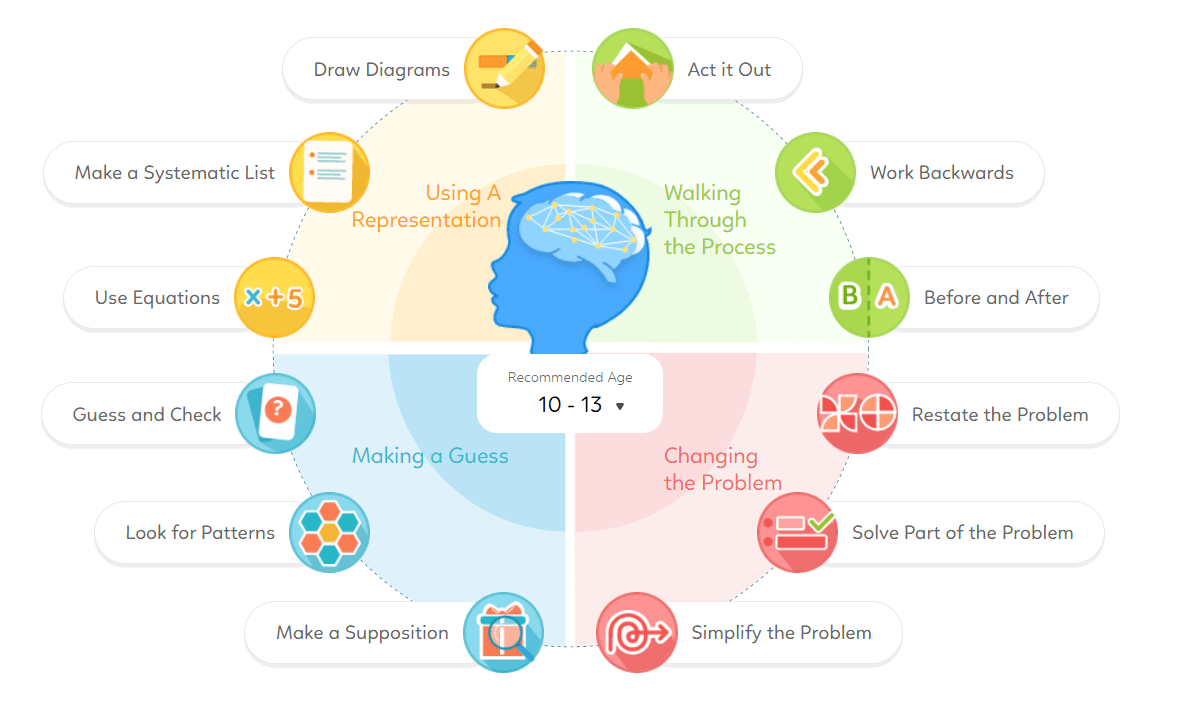Draw a Diagram
• Challenging - Whole Numbers - 3 quantities, 3 total amount of 2 quantities
• Intermediate - Fractions - Age, 2 quantities, amount of 1 quantity, 1 relationship
• Intermediate - Fractions - amount of 1 quantity, fraction of remainder, fraction of original left
• Challenging - Fractions - 2 or 3 quantities, fraction of 1 quantity, fraction of remainder, amount of 1 quantity
• Challenging - Fractions - Equal fractions, 2 quantities, 2 fractions, 1 comparison
• Challenging - Fractions - 2 or 3 events, fraction of remainder, amount of remainder, fraction + number
• Challenging - Fractions - 2 quantities, 1 relationship, everything changed, 1 comparison
• Intermediate - Ratio - 2 quantities, amount of each quantity, same amount change, 1 relationship
• Intermediate - Ratio - 2 quantities, 2 ratios, everything changed
• Challenging - Ratio - Double If, internal transfer, 2 quantities, 2 amount transferred, 2 ratios
• Intermediate - Average - Double Ifs, 2 quantities, 2 total averages, 2 comparisons
• Challenging - Percentage - 3 quantities, 1 relationship, 1 comparison, amount of 1 quantity
• Challenging - Percentage - 3 quantities, 2 percentage more/less, total amount
• Challenging - Percentage - 3 quantities, 2 relationships, 1 comparison
• Intermediate- Area of a triangle - longest side
• Challenging - Time - 2 quantities, total amount, 2 rates, different start time
• Basic - Pie Chart - fractions
• Basic - Algebra - 2 quantities, total amount, 1 comparison/ relationship
• Intermediate - Algebra - 2 quantities, amount of 1 quantity, 1 relationship/comparison
• Challenging - Algebra - 3 quantities, amount of 1 quantity, 1 relationship, 1 comparison
• Challenging - Algebra - 3 quantities, total amount, 2 comparisons
• Challenging - Rate - Same rate, 3 conditions
• Intermediate - Shapes - Maximum number of identical shapes
Make a Systematic Lis
• Intermediate - Whole Numbers - Common multiple, 2 quantities, fixed distance/ duration
• Intermediate - Whole Numbers - Sum of 2 numbers, number of ways
• Challenging - Whole Numbers - Common multiple, 3 quantities, fixed distance/ duration
• Challenging - Whole Numbers - 4 or more quantities, 1 condition
Use Equations
• Intermediate - Whole Numbers - Internal transfer, 2 quantities, 2 comparisons, fraction of amount transferred
• Intermediate - Whole Numbers - 2 quantities, 1 relationship, everything changed, 1 comparison
• Challenging - Fractions - 2 quantities, 2 relationships, everything changed
• Challenging - Percentage - 2 quantities, 2 or 3 percentages changed, total amount(s)
• Basic - Volume - finding length of a cube
Guess and Check
• Intermediate - Whole Numbers - Sum of 3 numbers, list of numbers
• Challenging - Whole Numbers - 2 quantities, 1 add & 1 subtract, total amount, amount of each quantity, total quantity
• Challenging - Whole Numbers - 3 quantities, 1 condition
• Intermediate - Percentage - 2 quantities, total amount, 1 relationship, amount of each quantity
• Intermediate - Average - total quantity, 1 comparison, total average, average of 1 quantity
Look for Patterns
• Intermediate - Whole Numbers - Figure Pattern
• Intermediate - Whole Numbers - Constant Change, starting amount, amount of change, find total sum
• Challenging - Whole Numbers - Starting amount, amount of change, amount of a particular day, find total sum
• Challenging - Whole Numbers - Number Pattern, fixed columns
Make a Supposition
• Intermediate - Whole Numbers - 2 quantities, total quantity, total amount, amount of each quantity
• Challenging - Whole Numbers - 2 quantities, 1 add & 1 subtract, total amount, total quantity, amount of each quantity
• Intermediate - Average - total quantity, 1 comparison, total amount, average of 1 quantity
• Intermediate - Average - total quantity, total amount, average of each quantity
Act it Out
• Intermediate - Shapes - 3D shapes
• Intermediate - Volume - Fitting/ cutting smaller cuboids into/ from a larger cuboid
• Basic - Nets - Missing faces
Work Backwards
• Intermediate - Whole Numbers - Internal transfer, 2 quantities, 2 events, amounts transferred
• Intermediate - Fractions - Equal Stage (end), internal transfer, 2 quantities, total amount, 2 events, 2 fractions of amount transferred
• Intermediate - Percentage - 2 quantities, total amount, 1 comparison, discount
• Challenging - Percentage - 2 quantities, 3 events, 1 relationship, final amount for each quantity
• Challenging - Algebra - 2 quantities, 2 events, 2 relationships, amount of each quantity (end)
Use Before and After Concept
• Basic - Whole Numbers - Constant difference, age
• Challenging - Whole Numbers - Equal Stage (end), 3 or more quantities, total amount, more than 3 events
• Intermediate - Fractions - 2 quantities, 2 relationships, amount of total/1 quantity
• Challenging - Fractions - Internal transfer, 2 quantities, 2 comparison, fraction of amount transferred
• Intermediate - Ratio - Constant difference, 2 quantities, equal decrease/increase, 2 relationships, 1 amount
• Challenging - Ratio - 3 quantities, 2 relationships, amount of total/1 quantity
Restate the Problem
• Challenging - Whole Numbers - Sum and difference of a sequence of numbers
• Challenging - Whole Numbers - 3 quantities, 3 different characteristics, overlap in characteristics, total quantity
• Intermediate - Angles - Angles in composite figures
• Challenging - Area & Perimeter - Fraction of the figure shaded, 1 relationship
Solve Part of the Problem
• Challenging - Percentage - 2 shapes, overlap, find percentage of composite figure shaded
• Challenging - Rate - 3 quantities, rates of different categories
• Intermediate - Area of Triangle - composite figure, shaded area
• Intermediate - Area of Triangle - Common height
• Intermediate - Angles - 2 shapes, overlap
Simplify the Problem
• Intermediate - Whole Numbers - 2 quantities, equal amount, total amount, amount of each quantity
• Intermediate - Whole Numbers - amount of 1 quantity, fraction of remainder, fraction of original left
• Basic - Ratio - 2 quantities, 1 relationship, difference in quantity/ total quantity
• Challenging - Ratio - Constant quantity, 3 quantities, 2 ratios, amount of total/1 quantity
• Basic - Area & Perimeter - 1 type of shape, 2 lengths

## The Maths Platform Children Love to Use

Where Learning Comes to Life

Higher-Order Thinking Skills

The Ultimate Challenge for Maths Mastery

Top Schools’ Questions in One Place

Support
Resources
Let's Connect